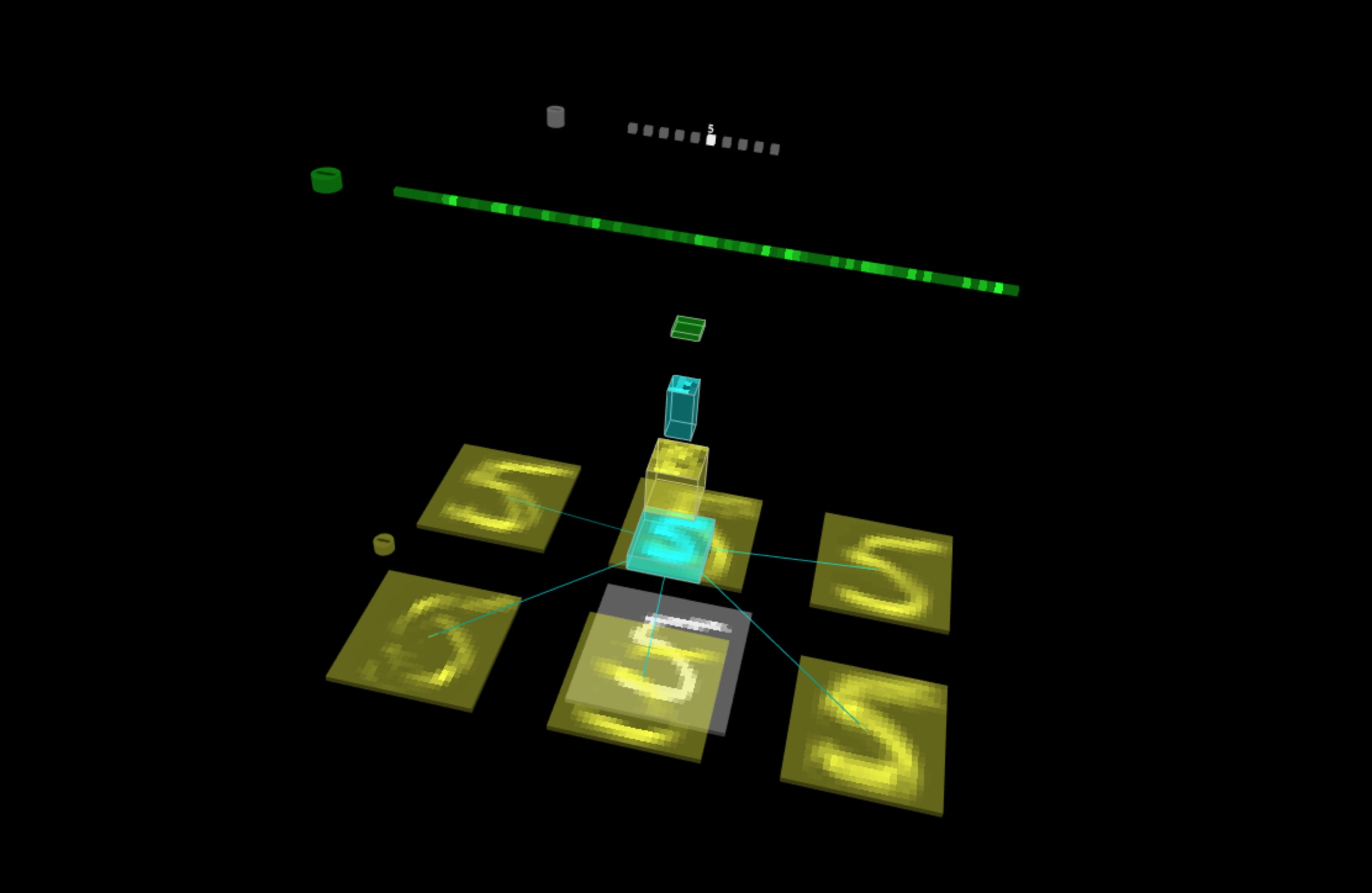TensorSpace.jsTensorSpace Converter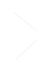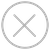filter_center_focus TensorSpace 可视化代码

tf.keras 模型

``````\$ tensorspacejs_converter \
--input_model_from="tensorflow" \
--input_model_format="tf_keras" \
--output_layer_names="conv_1,maxpool_1,conv_2,maxpool_2,dense_1,dense_2,softmax" \
./rawModel/keras/tf_keras_model.h5 \
./convertedModel/layerModel``````
wb_sunny注意:
• filter_center_focusinput_model_from 设置成 tensorflow
• filter_center_focusinput_model_format 设置成 tf_keras
• filter_center_focus.h5 文件的路径设置到 input_path 中。
• filter_center_focus 取出 tf.keras 模型的 Layer 名称 , 然后设置到 output_layer_names 中，如 图1 所示。
• filter_center_focus 以上 TensorSpace-Converter 预处理脚本将会在 convertedModel/layerModel 文件夹中生成经过预处理的模型。在本教程中，我们已经生成了经过预处理的模型，模型文件可以在 这个文件夹 中找到。
tf.keras 模型 (结构权重分开保存)

``````\$ tensorspacejs_converter \
--input_model_from="tensorflow" \
--input_model_format="tf_keras_separated" \
--output_layer_names="Conv2D_1,MaxPooling2D_1,Conv2D_2,MaxPooling2D_2,Dense_1,Dense_2,Softmax" \
./rawModel/keras_separated/topology.json,./rawModel/keras_separated/weights.h5 \
./convertedModel/layerModel``````
wb_sunny注意:
• filter_center_focusinput_model_from 设置成 tensorflow
• filter_center_focusinput_model_format 设置成 tf_keras_separated
• filter_center_focus 对于这种模型类型，因为有两个模型文件，在设置 TensorSpace-Converter 的 input_path 时，合并两个文件的路径，并用英文半角逗号“,”分开，将 .json 文件的路径在前，.h5 文件的路径在后。
• filter_center_focus 取出 tf.keras 模型的 Layer 名称 , 然后设置到 output_layer_names 中，如 图1 所示。
• filter_center_focus 以上 TensorSpace-Converter 预处理脚本将会在 convertedModel/layerModel 文件夹中生成经过预处理的模型。在本教程中，我们已经生成了经过预处理的模型，模型文件可以在 这个文件夹 中找到。
frozen 模型

``````\$ tensorspacejs_converter \
--input_model_from="tensorflow" \
--input_model_format="tf_frozen" \
--output_layer_names="MyConv2D_1,MyMaxPooling2D_1,MyConv2D_2,MyMaxPooling2D_2,MyDense_1,MyDense_2,MySoftMax" \
./rawModel/frozen/frozen_model.pb \
./convertedModel/graphModel``````
wb_sunny注意:
• filter_center_focusinput_model_from 设置成 tensorflow
• filter_center_focusinput_model_format 设置成 tf_frozen
• filter_center_focus.pb 文件的路径设置到 input_path 中。
• filter_center_focus 取出 frozen 模型的 Node 名称 , 然后设置到 output_layer_names 中，如 图2 所示。
• filter_center_focus 以上 TensorSpace-Converter 预处理脚本将会在 convertedModel/graphModel 文件夹中生成经过预处理的模型。在本教程中，我们已经生成了经过预处理的模型，模型文件可以在 这个文件夹 中找到。
tf.keras 模型 (结构权重分开保存)

``````\$ tensorspacejs_converter \
--input_model_from="tensorflow" \
--input_model_format="tf_saved" \
--output_layer_names="MyConv2D_1,MyMaxPooling2D_1,MyConv2D_2,MyMaxPooling2D_2,MyDense_1,MyDense_2,MySoftMax" \
./rawModel/saved \
./convertedModel/graphModel``````
wb_sunny注意:
• filter_center_focusinput_model_from 设置成 tensorflow
• filter_center_focusinput_model_format 设置成 tf_saved
• filter_center_focus 将 saved 模型文件夹路径设置到 input_path 中。
• filter_center_focus 取出 saved 模型的 Node 名称 , 然后设置到 output_layer_names 中，如 图2 所示。
• filter_center_focus 以上 TensorSpace-Converter 预处理脚本将会在 convertedModel/graphModel 文件夹中生成经过预处理的模型。在本教程中，我们已经生成了经过预处理的模型，模型文件可以在 这个文件夹 中找到。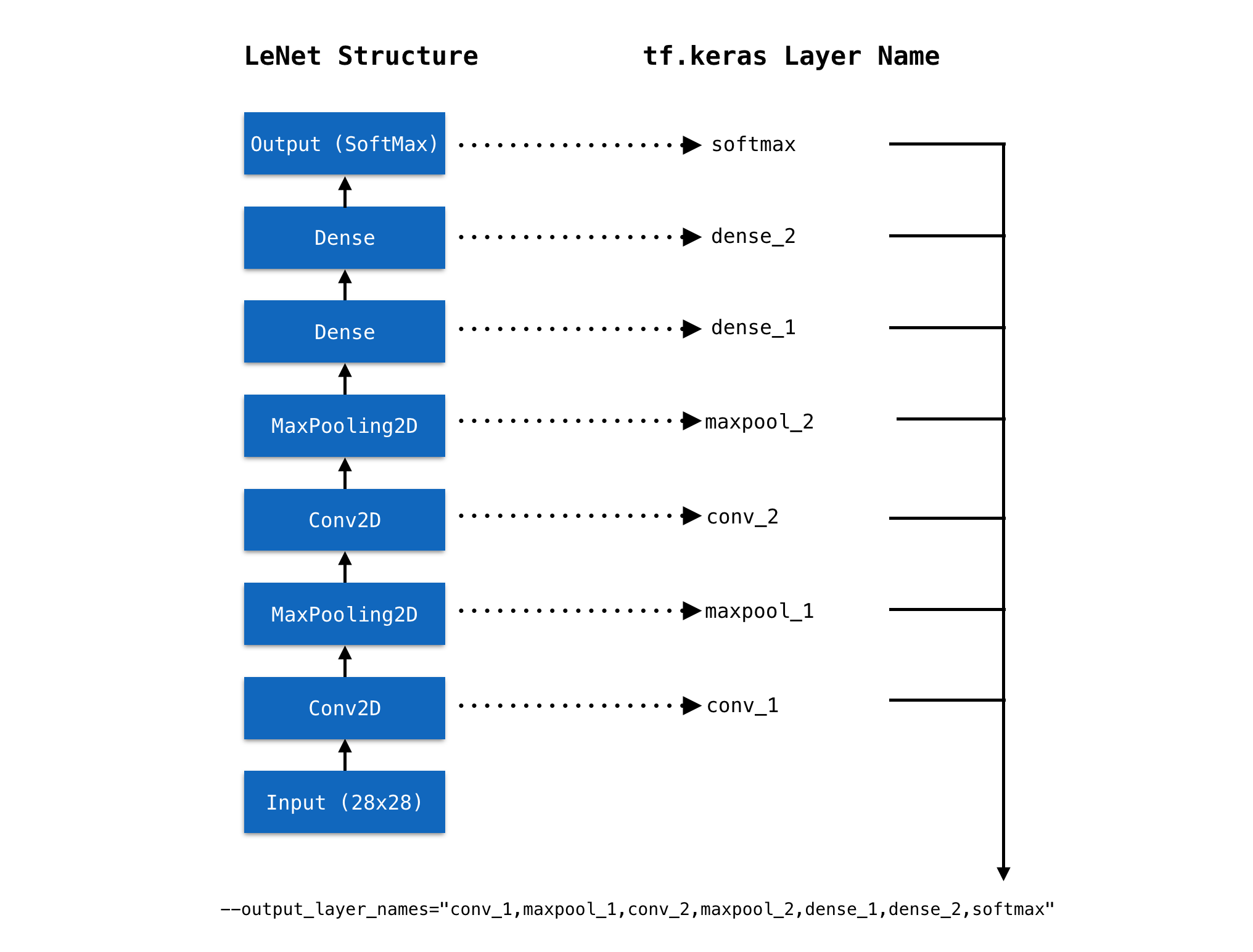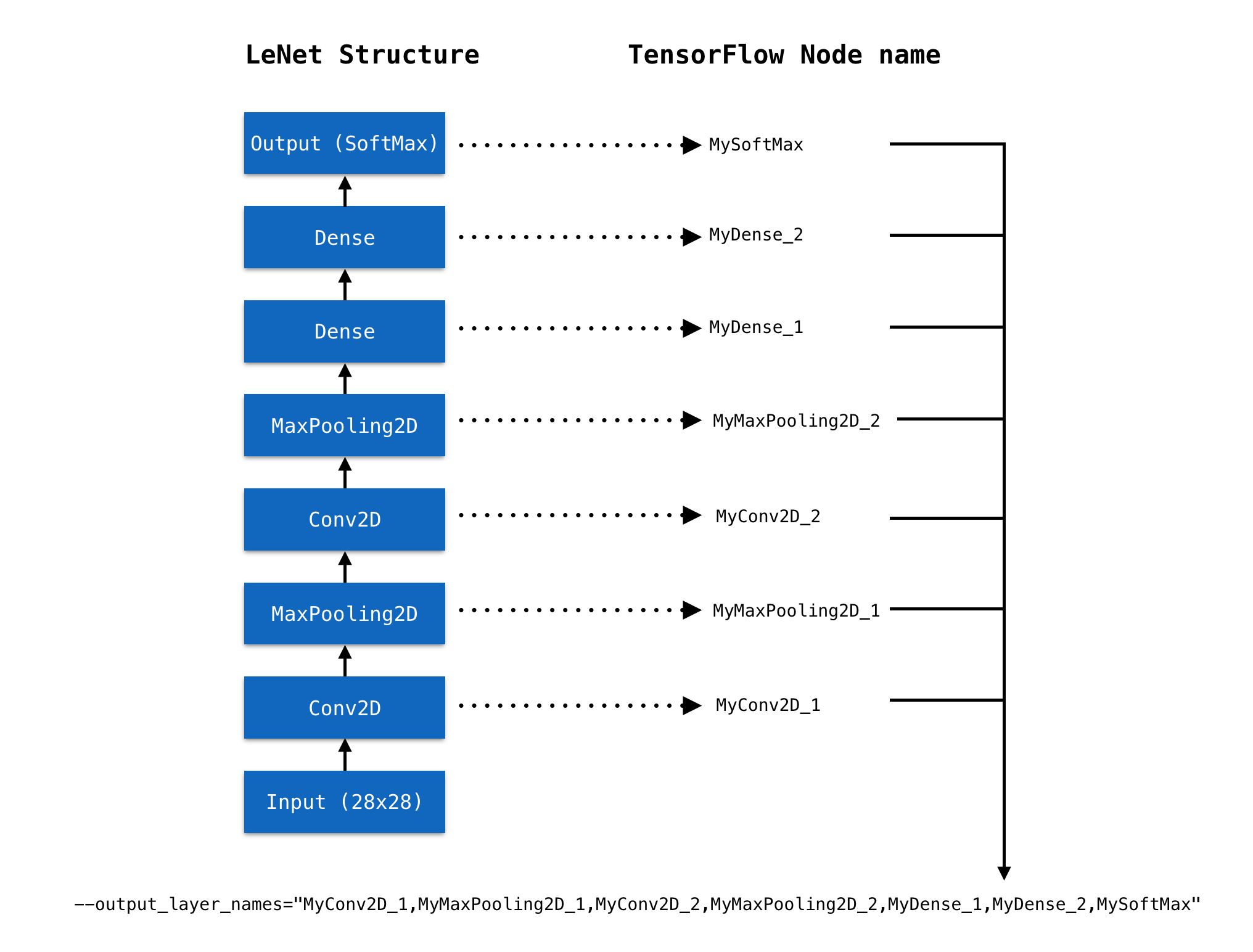wb_sunny注意:
• filter_center_focus 我们将会得到2种不同类型的文件：
• flare 一份 model.json 文件：包含所得到的模型结构信息（包括中间层输出）。
• flare 一些 权重 文件：包含模型训练所得到的权重信息。权重文件的数量取决于模型的结构。

``````let model = new TSP.models.Sequential( modelContainer );

outputs: [ "0", "1", "2", "3", "4", "5", "6", "7", "8", "9" ]
} ) );``````
TensorSpace 在载入模型时，对于不同种类的 TensorFlow 模型，需要进行不同的配置。
filter_center_focus 对于 TensorSpace-Converter 生成的 layer 模型 (tf.keras 模型)：
``````model.load( {
type: "tensorflow",
url: "./convertedModel/layerModel/model.json"
} );``````
filter_center_focus 对于 TensorSpace-Converter 生成的 graph 模型 (tfrozen 模型或者 saved 模型)，需要配置 TensorSpace Loader 的 outputsName 属性，属性中的name 和在使用 TensorSpace-Converter 转化时输入的 output_layer_names 相同：
``````model.load( {
type: "tensorflow",
url: "./convertedModel/graphModel/model.json",
outputsName: ["MyConv2D_1", "MyMaxPooling2D_1", "MyConv2D_2", "MyMaxPooling2D_2", "MyDense_1", "MyDense_2", "MySoftMax"]
} );``````